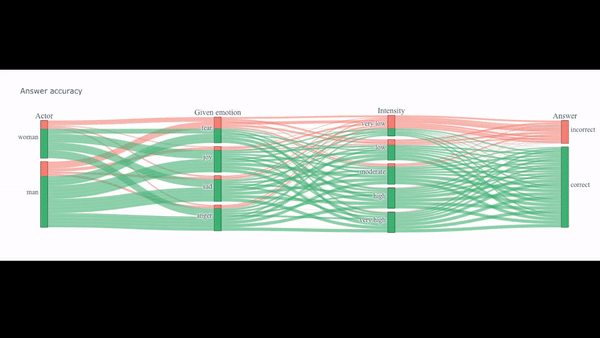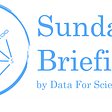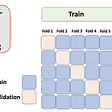# Aim

Building machine learning models that produce high accuracy is getting easier, but when it comes to interpretability, most of them are still far from good. In many cases, you might need to put more emphasis on understanding the models than accuracy.

As a powerful yet simple technique, generalized additive model (GAM) is underrepresented. Only few people know apply it in their daily work. To understand how GAM works in R. I have performed method like lm() function for linear regression and gam() function. Firstly, lets understand what is exactly GAMs.

## Introduction

Whenever we build statistical models, we face a trade-off between flexibility and interpretability. GAMs offer simple solution between models, such as those who fit with linear regression, and more complex machine learning models like neural networks. Linear models are easy to interpret and easy to derive inference; However, we often need to model more complex phenomena that can be represented by linear relationships.

On other hand, machine learning models, like boosted trees or neural networks, can be very good at making predictions of complex relationships. The problem is that they need lots of data, are quite difficult to interpret, and one can rarely make inference from model results.

GAMs offer; they can fit to complex models, nonlinear relationships and make good predications in these cases. Let’s try to understand how actually do they work.

The purpose of GAMs is to maximize the quality of the prediction of a dependent variable Y from various distributions, by specific non-parametric functions of the predictor variable which are connected to dependent variable via a link function. The structure of GAM can be written as:

1. g(E(Y)) is the link function that links the expected value to the predictor variables x1,x2,…,xm.
2. f1(x1) + f2(x2) + … +fm(xm) is the functional form with an additive series. Response variable Y.

How GAMs Works ?

Plotted below a Non-Linear Relationship in which Linear Model is fitted. Used lm() function. The model doesn’t capture key points of relationship.

With GAM, we can fit data with smooths, or splines, which are functions that can take on a wide variety of shapes. Here, used mgcv library and gam() function

## Stepwise Approach for using GAM:

a. GAM is applied on datasets having non-linear relationship among dependent & independent variables.

c. Gam can fit models for standard distributions like binomial, gamma, Gaussian and Poisson.

d. Select spline term: degree of freedom, initial smoothing parameter, so on..

e. Model package: mgcv, gam

## Implementation in R!

We will use the `gam()` function in R to fit a GAM.

The s() function, which is part of the gam library, is used to indicate that s() we would like to use a smoothing spline. We specify that the function of year and age should have 6 degrees of freedom. Since education is qualitative, we leave it as is, and it is converted into four dummy variables.

## Results

In the image has 3 different plots for each variable included in the Model. The X-axis contains age, year, education and the Y-axis contains the Response values i.e the Salaries.

From the plots, we can see that Salary first increases with ‘age’ then decreases after around 60. For variable ‘year’ the Salaries tend to increase, and it seems that there is a decrease in salary at around year 2007 or 2008. And for the Categorical variable ‘education’, Salary also increases.

The curvy shapes for the variables ‘age’ and ‘year’ is due to the Smoothing splines which models the Non linearities in the data. The dotted Lines around the main curve lines are the Standard error.

Hence this is a very effective way of fitting Non-linear functions on several variables and producing the plots for each and study the effect on the Response.

Fitting a GAM with a smoothing spline is not quite as simple as fitting a GAM with a natural spline, since in the case of smoothing splines, least squares cannot be used. However, standard software such as the gam() function in R can be used to fit GAMs using smoothing splines, via an approach known as “backfitting Algorithm”.

The basic idea in Splines is that we are going to fit Smooth Non-linear Functions on a bunch of Predictors Xi to capture and learn the Non-linear relationships between the Model’s variables i.e X and Y

GAM is based on ‘smoothers.’ Broadly, it can be classified into three types:

(i) Regression splines (B-spline, P-spline): These are commonly used because of easy computation, and it can be written as a linear combination of basis function; which is well suited for estimation and prediction.

(ii) Local regression (loess): It’s based on nearest neighborhood-based smoothers class. Local regression adopts the approach based on running mean smoothers. Loess produces a smoother curve than the running mean by fitting a weighted regression within each nearest-neighbor window.

(iii) Smoothing Splines: It estimates the smooth function by minimizing the penalized sum of squares. The major drawback of smoothing spline is number effective degrees of freedom used which are much less than the number of knots (due to the penalty).

## Conclusion

Generalized Additive Models are very nice and effective way of fitting Linear Models which depends on some smooth and flexible Non-linear functions fitted on some predictors to capture Non-linear relationships in the data. We can also, take model having non-linear data and can perform gam and compare with ANOVA which will tell the goodness of fit in the different models. GAMs are additive in nature, which means there are no interaction terms in the Model.

Thanks a lot for reading the article, and make sure to like and share it. Cheers!

--

--

--

## More from The Startup

Get smarter at building your thing. Follow to join The Startup’s +8 million monthly readers & +756K followers.

## Cracking The Zed Run Code Part 9b (The Secret to Selecting the Right Race, Mane Event!)## How to say No to Data Science Projects?## Beginning with Ourselves## From Prediction to Action — How to Learn Optimal Policies From Data (Part 1)## Glove Research Paper Clearly Explained## scarlet fever Market Analysis by Capital Investment, Industry Outlook, Growth Potential…Data Scientist

## The science behind the data N°3 —  The advantages of Parallel Categorical Diagrams## The Future of Statistical Computing Environment Platforms## D4S Sunday Briefing #148## Target Encoding: things you must consider